# ISEE Middle Level Quantitative : How to find the square root

## Example Questions

← Previous 1 3

### Example Question #1 : How To Find The Square Root

Give the square root of 256.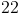Explanation: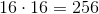- that is, 16 squared is 256, making 16 the square root of 256 by definition.

### Example Question #2 : How To Find The Square Root

Which is the greater quantity?

(a)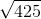(b)(a) and (b) are equal

It is impossible to tell from the information given

(b) is greater

(a) is greater

(b) is greater

Explanation: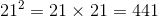, so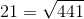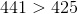, so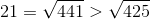### Example Question #3 : How To Find The Square Root

Which is the greater quantity?

(a)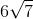(b)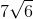It is impossible to tell from the information given

(a) is greater

(b) is greater

(a) and (b) are equal

(b) is greater

Explanation:

(a)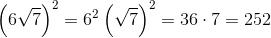, so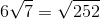(b)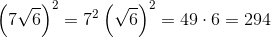, so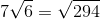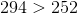,

so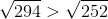,

and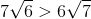### Example Question #3 : How To Find The Square Root

Column A     Column B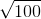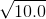There is no relationship between the quantities.

The quantity in Column A is greater.

The quantity in Column B is greater.

The quantities are equal.

The quantity in Column A is greater.

Explanation:

The square root of 100 is 10, while the square root of 10 is between the square root of 9 and 16, so about 4. Therefore, Column A has to be greater.

### Example Question #4 : How To Find The Square Root

Which of the following is equal to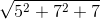?None of the other responses is correct.Explanation: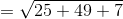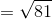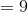### Example Question #6 : How To Find The Square Root

Which of the following is equal to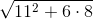?None of the other responses is correct.Explanation: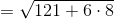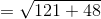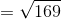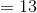### Example Question #5 : How To Find The Square Root

Which of the following is equal to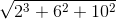?None of the other responses is correct.Explanation: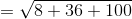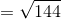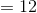### Example Question #8 : How To Find The Square Root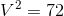is positive.

Which is the greater quantity?

(a)(b)It is impossible to tell which is greater from the information given

(B) is greater

(A) is greater

(A) and (B) are equal

(A) is greater

Explanation:

Sinceis positive, we can compareto 8 by comparing their squares.and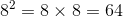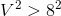, so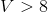, making (A) greater.

### Example Question #6 : How To Find The Square Root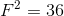Which is the greater quantity?

(A)(B)(A) is greater

(B) is greater

(A) and (B) are equal

It is impossible to tell which is greater from the information given

It is impossible to tell which is greater from the information given

Explanation:

If, then one of two things is true - either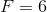or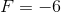.

However,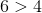and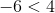, so it is impossibe to tell whether (A) or (B) is greater.

### Example Question #7 : How To Find The Square Root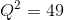Which is the greater quantity?

(A)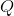(B)(A) is greater

(B) is greater

It is impossible to tell which is greater from the information given

(A) and (B) are equal

If, then one of two things is true - either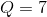or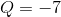. Since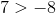and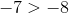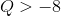either way, so (A) is greater.